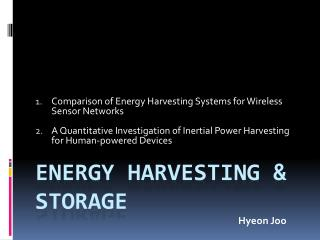Download PresentationENERGY HARVESTING & storage

# ENERGY HARVESTING & storage - PowerPoint PPT PresentationDownload Presentation## ENERGY HARVESTING & storage

- - - - - - - - - - - - - - - - - - - - - - - - - - - E N D - - - - - - - - - - - - - - - - - - - - - - - - - - -
##### Presentation Transcript

1. Comparison of Energy Harvesting Systems for Wireless Sensor Networks A Quantitative Investigation of Inertial Power Harvesting for Human-powered Devices ENERGY HARVESTING & storage HyeonJoo

2. OUTLINE • Power requirement • Suitable scavenging energy source • Energy conversion device • Vibration

3. Power requirement

4. Power requirement

5. Power requirement Actuator and Communication consumes a large proportion of total power

6. Suitable scavenging energy source

7. Mechanical energy source • Steady state mechanical source • Steady state: wind, flow, current.. • Intermittent mechanical source • Human activity(walking, typing.. 5.88J/2steps ) • Vehicles passing • Vibration • Energy depends on the amplitude and its freq. • Mass of harvesting device relative to the vibrating mass

8. Mechanical energy source Typically vibration is made up of a number of fundamental freq. and their harmonic

9. Energy conversion devices

10. Vibration m: seismic mass k: spring of stiffness u(t): Position of case x(t): Position of the seismic b: Damping coefficient

11. Vibration m: seismic mass k: spring of stiffness u(t): Position of case x(t): Position of the seismic b: Damping coefficient Mx’’(t) + Bx’(t) + Kx(t) = - Mu’’(t)

12. Vibration m: seismic mass k: spring of stiffness u(t): Position of case x(t): Position of the seismic b: Damping coefficient

13. Vibration mx’’(t) + bx’(t) + kx(t) = - mu’’(t)

14. Vibration ω : vibration freq ωn : natural freq : damping factor ω =ωn => peak power (resonant)

15. Vibration ω : vibration freq ωn : natural freq : damping factor • Increase the damping factor • Reducing the peak power, • but increase bw

16. Vibration ω : vibration freq ωn : natural freq : damping factor Thus, fixed freq => low d.f. Various freq => high d.f.

17. Vibration • Harvested energy is proportional to the m

18. Vibration • Piezoelectric conversion (pressure) • Commonly used material: PZT, BaTiO3, PVDF • Electrostatic conversion • The formation of a parallel plate capacitor • Electromagnetic conversion • Magnetic and coil

19. Intermittent mechanical conv. • Piezoelectric conversion • Electro-active polymers(EAP) conversion • Electromagnetic conversion

20. Any Questions?

21. OUTLINE • Motivation • Mobile device • Energy harvesting model • Data Analysis • Power Estimation

22. Motivation • Human motion energy harvesting • Electronic devices • Realistic experiment • 6 different parts of body

23. Mobile devices Wearable device Wearable device

24. Energy harvesting model k: spring constant m:proof mass d: damping coefficient y(t): generator displacement z(t): generator’s motion Zmax: interval travel limit

25. Energy harvesting model • Loggers: 1GB SD 80HZ

26. Data Analysis • 3-aixs accelerometers (X,Y,Z) • Harvest energy from daily human activities using free motion • Only kinetic energy from human body • Zero-gravity for accuracy • High-passed filtered with 0.05Hz cuffoff

27. Data Analysis

28. Data Analysis

29. Data Analysis

30. Any questions?